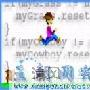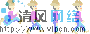# 關于文曲星上猜數字遊戲的c編程方法

#include<stdio.h>

#include<stdlib.h>

#include<time.h>

#include<math.h>

#define SIZE 4

main()

{

int enter=1,stop,a[SIZE],b[SIZE],guessnum,i,j,counter,countera,counterb,counter0,extra;

char ch;

srand(time(NULL));

while(enter==1)

{ stop=2;

while(stop==2)

{ extra=1;

for(i=0;i

a[i]=rand()%10;

for(i=0;i

{ for(j=i+1;j

{ if(a[i]==a[j]) extra=2; }

}

if(a!=0&&extra==1) {stop=1;PRintf("%d%d%d%d",a,a,a,a);}

}

counter=1;

while(counter

scanf("%d",&guessnum);

for(i=0;i

b[i]=guessnum/(int)pow(10,SIZE-1-i)%10;

countera=0;

for(i=0;i

{ if(a[i]==b[i]) countera++;}

counter0=0;

for(i=0;i

{ for(j=0;j

{if(a[i]==b[j]) counter0++;}

}

counterb=(counter0-countera);

if(countera==4) {printf("Wonderful!You guessed the number.Then,please input your choice,'1' represents going on,'2' represents breaking\n");

counter=7;

scanf("%d",&enter);

}

else {printf("%dA%dB\n",countera,counterb);

counter++;

}

}

}

while((ch=getchar())!='s'&&ch!='S');

return 0;

}Java多線程編程基礎之非線程的方法
[wait(),notify()/notityAll()方法] 　　關于這兩個方法,有很多的內容需要說明.在下面的說明中可能會有很多地方不能一下子明白,但在看完本節後,即使不能完全明白,你也一定要回過頭來記住下面的兩句話:　　[wait(),...查看完整版>>Java多線程編程基礎之非線程的方法

Java遊戲編程初步現在流行的遊戲似乎都是用C或C++來開發的。在java平台上幾乎沒有很大型及可玩的流行遊戲。由于java是個新生語言，他的許多特性還有待大家的發掘,但是我們不能否認Java在遊戲編程方面的強大性。本文將帶領大家一步...查看完整版>>Java遊戲編程初步

Java遊戲編程讀書筆記GameCanvas實際上就是屏幕上一個可繪制區域. Javax.microedition.lcdui.game.GameCanvas 類與javax.microedition.lcdui.Canvas 有以下兩點不一樣: 圖像緩沖以及可以查詢按鍵的狀態. 這些改進給遊戲開發者更多的便利...查看完整版>>一步一步學習midp2。0遊戲編程（二）